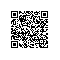# 自动建立正确索引(btree,hash,gin,gist,sp-gist,brin,bitmap...)的方法

### 标签

PostgreSQL , 索引接口 , 自动创建索引 , 自动选择索引接口 , (btree,hash,bitmap,gin,gist,sp-gist,brin,rum,bloom,zoomdb)

## 背景

PostgreSQL的索引接口是开放的，支持btree,hash,bitmap,gin,gist,sp-gist,brin,rum,bloom,zoomdb等索引接口。因此，不同的数据类型，有不同的索引结构可以选择。

《懒人推动社会进步 - 多列聚合, gin与数据分布(选择性)》

## 语法

CREATE [ UNIQUE ] INDEX [ CONCURRENTLY ] [ [ IF NOT EXISTS ] name ] ON table_name [ USING method ]
( { column_name | ( expression ) } [ COLLATE collation ] [ opclass ] [ ASC | DESC ] [ NULLS { FIRST | LAST } ] [, ...] )
[ WITH ( storage_parameter = value [, ... ] ) ]
[ TABLESPACE tablespace_name ]
[ WHERE predicate ]


## 索引选择的要素

### 必要约束

1、如果用户要创建 unique 索引，那么只能选择btree索引接口。

2、某些类型指支持某类索引接口，例如数组类型、全文检索类型，只支持GIN索引。GIS类型只支持gist或sp-gist索引。

### 选择性

1、选择性差（例如1亿记录，有100-10万 条唯一值），建议使用gin或bitmap索引。

2、选择性好（例如1亿记录，有8000万唯一值），建议使用btree或hash索引。

### 数据分布

1、对于数据值与行号呈现较强的线性相关特性时，加入用户没有按该列顺序输出的需求。则建议使用brin块级索引。

2、当列长度超过数据块的1/3时，不能使用btree，建议使用hash索引。或者使用表达式btree索引，建少索引entry的大小。

### 查询需求

1、范围查询、排序查询、等值查询

2、仅仅有等值查询

3、有多个列的任意组合查询需求

4、有包含、相交等查询需求

5、有距离、距离排序、相交、包含、贯穿等查询需求

### 小结

pg_class.reltuples，行数

pg_stats.n_distinct，唯一值个数或比例

pg_stats.correlation，列的线性相关性


## 例子

### Greenplum

Command:     CREATE INDEX
Description: define a new index
Syntax:
CREATE [UNIQUE] INDEX name ON table
[USING btree|bitmap|gist]
( {column | (expression)} [opclass] [, ...] )
[ WITH ( FILLFACTOR = value ) ]
[TABLESPACE tablespace]
[WHERE predicate]


#### UDF

create or replace function build_idx(
sch name, -- schema name
tbl name, -- 表名
col name, -- 列名
idx name -- 索引名
) returns void as
$$declare v_tuples numeric; v_n_distinct_cnt numeric; v_n_distinct numeric; v_corr numeric; v_sql text; am text := 'btree'; begin -- 建议先收集统计信息, 或者根据统计信息的状态，判断是否要更新统计信息 select reltuples into v_tuples from pg_class where relname=tbl and relnamespace=(select oid from pg_namespace where nspname=sch); -- 唯一值个数，100 ~ 10万 , 同时唯一值比例低于1/10000，使用bitmap(gp) , 或者使用gin(pg) select case when n_distinct >=1 then n_distinct when n_distinct=-1 then v_tuples else abs(n_distinct*v_tuples) end into v_n_distinct_cnt from pg_stats where schemaname=sch and tablename=tbl and attname=col; -- 唯一值比例，低于1/10000 ，使用bitmap(gp) , 或者使用gin(pg)。否则使用btree select case when n_distinct >=1 then n_distinct/v_tuples when n_distinct=-1 then 1 else abs(n_distinct) end into v_n_distinct from pg_stats where schemaname=sch and tablename=tbl and attname=col; if v_n_distinct <= 1/10000.0 and v_n_distinct_cnt between 100 and 100000 then am = 'bitmap'; end if; v_sql := 'create index '||quote_ident(idx)||' on '||quote_ident(sch)||'.'||quote_ident(tbl)||' using '||am||' ('||quote_ident(col)||')'; raise notice '%', v_sql; execute v_sql; end;$$ language plpgsql strict;


postgres=# create table tbl(id int, info text);
NOTICE:  Table doesn't have 'DISTRIBUTED BY' clause -- Using column named 'id' as the Greenplum Database data distribution key for this table.
HINT:  The 'DISTRIBUTED BY' clause determines the distribution of data. Make sure column(s) chosen are the optimal data distribution key to minimize skew.
CREATE TABLE
postgres=# insert into tbl select 200*random(), 'test' from generate_series(1,3000000);
INSERT 0 3000000
postgres=# select build_idx('public','tbl','id','test_idx_id');
NOTICE:  create index test_idx_id on public.tbl using bitmap (id)
build_idx
-----------

(1 row)


### PostgreSQL

Command:     CREATE INDEX
Description: define a new index
Syntax:
CREATE [ UNIQUE ] INDEX [ CONCURRENTLY ] [ [ IF NOT EXISTS ] name ] ON table_name [ USING method ]
( { column_name | ( expression ) } [ COLLATE collation ] [ opclass ] [ ASC | DESC ] [ NULLS { FIRST | LAST } ] [, ...] )
[ WITH ( storage_parameter = value [, ... ] ) ]
[ TABLESPACE tablespace_name ]
[ WHERE predicate ]


#### UDF

create or replace function build_idx(
sch name, -- schema name
tbl name, -- 表名
col name, -- 列名
idx name -- 索引名
) returns void as
$$declare v_tuples numeric; v_n_distinct_cnt numeric; v_n_distinct numeric; v_corr numeric; v_sql text; am text := 'btree'; begin -- 建议先收集统计信息, 或者根据统计信息的状态，判断是否要更新统计信息 select reltuples into v_tuples from pg_class where relname=tbl and relnamespace=sch::regnamespace; -- 唯一值个数，100 ~ 10万 , 同时唯一值比例低于1/10000，使用bitmap(gp) , 或者使用gin(pg) select case when n_distinct >=1 then n_distinct when n_distinct=-1 then v_tuples else abs(n_distinct*v_tuples) end into v_n_distinct_cnt from pg_stats where schemaname=sch and tablename=tbl and attname=col; -- 唯一值比例，低于1/10000 ，使用bitmap(gp) , 或者使用gin(pg)。否则使用btree select case when n_distinct >=1 then n_distinct/v_tuples when n_distinct=-1 then 1 else abs(n_distinct) end into v_n_distinct from pg_stats where schemaname=sch and tablename=tbl and attname=col; -- 相关性, abs>0.9 使用brin select abs(correlation) into v_corr from pg_stats where schemaname=sch and tablename=tbl and attname=col; if v_corr>=0.9 then am = 'brin'; elsif v_n_distinct <= 1/10000.0 and v_n_distinct_cnt between 100 and 100000 then am = 'gin'; end if; -- 如何列大小超过1000字节，am='hash' v_sql := format('create index %I on %I.%I using '||am||' (%I)', idx, sch, tbl, col); raise notice '%', v_sql; execute v_sql; end;$$ language plpgsql strict;


postgres=# create table test(id int, info text);
CREATE TABLE
postgres=# insert into test select 1, 'test' from generate_series(1,1000000);
INSERT 0 1000000
postgres=# analyze test;
ANALYZE
postgres=# select build_idx('public','test','id','test_id_idx1212');
NOTICE:  00000: create index test_id_idx1212 on public.test using brin (id)
LOCATION:  exec_stmt_raise, pl_exec.c:3337
build_idx
-----------

(1 row)


## 延伸使用钉钉扫一扫加入圈子
+ 订阅